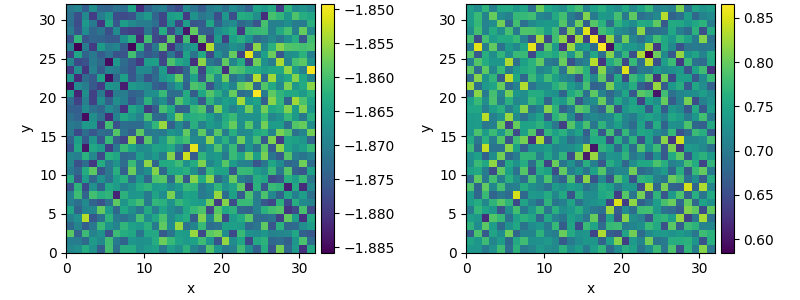# 2.20. Custom Class for coupled PDEs

This example shows how to solve a set of coupled PDEs, the spatially coupled FitzHugh–Nagumo model, which is a simple model for the excitable dynamics of coupled Neurons:

$\begin{split}\partial_t u &= \nabla^2 u + u (u - \alpha) (1 - u) + w \\ \partial_t w &= \epsilon u\end{split}$

Here, $$\alpha$$ denotes the external stimulus and $$\epsilon$$ defines the recovery time scale. We implement this as a custom PDE class below.0%|          | 0/100.0 [00:00<?, ?it/s]
Initializing:   0%|          | 0/100.0 [00:00<?, ?it/s]
0%|          | 0/100.0 [00:00<?, ?it/s]
0%|          | 0.24/100.0 [00:00<01:36,  1.03it/s]
1%|          | 0.73/100.0 [00:00<00:38,  2.58it/s]
3%|2         | 2.92/100.0 [00:00<00:16,  5.94it/s]
8%|7         | 7.71/100.0 [00:00<00:11,  8.17it/s]
15%|#4        | 14.85/100.0 [00:01<00:09,  8.68it/s]
23%|##2       | 22.98/100.0 [00:02<00:08,  8.83it/s]
32%|###1      | 31.61/100.0 [00:03<00:07,  9.23it/s]
41%|####1     | 41.12/100.0 [00:04<00:06,  9.41it/s]
51%|#####     | 50.89/100.0 [00:05<00:05,  9.34it/s]
60%|######    | 60.32/100.0 [00:06<00:04,  9.50it/s]
70%|#######   | 70.22/100.0 [00:07<00:03,  9.56it/s]
80%|########  | 80.17/100.0 [00:08<00:02,  9.51it/s]
90%|########9 | 89.7/100.0 [00:09<00:01,  9.60it/s]
100%|#########9| 99.7/100.0 [00:10<00:00,  9.63it/s]
100%|#########9| 99.7/100.0 [00:10<00:00,  9.60it/s]
100%|##########| 100.0/100.0 [00:10<00:00,  9.63it/s]
100%|##########| 100.0/100.0 [00:10<00:00,  9.63it/s]


from pde import FieldCollection, PDEBase, UnitGrid

class FitzhughNagumoPDE(PDEBase):
"""FitzHugh–Nagumo model with diffusive coupling"""

def __init__(self, stimulus=0.5, τ=10, a=0, b=0, bc="auto_periodic_neumann"):
super().__init__()
self.bc = bc
self.stimulus = stimulus
self.τ = τ
self.a = a
self.b = b

def evolution_rate(self, state, t=0):
v, w = state  # membrane potential and recovery variable

v_t = v.laplace(bc=self.bc) + v - v**3 / 3 - w + self.stimulus
w_t = (v + self.a - self.b * w) / self.τ

return FieldCollection([v_t, w_t])

grid = UnitGrid([32, 32])
state = FieldCollection.scalar_random_uniform(2, grid)

eq = FitzhughNagumoPDE()
result = eq.solve(state, t_range=100, dt=0.01)
result.plot()


Total running time of the script: ( 0 minutes 10.830 seconds)